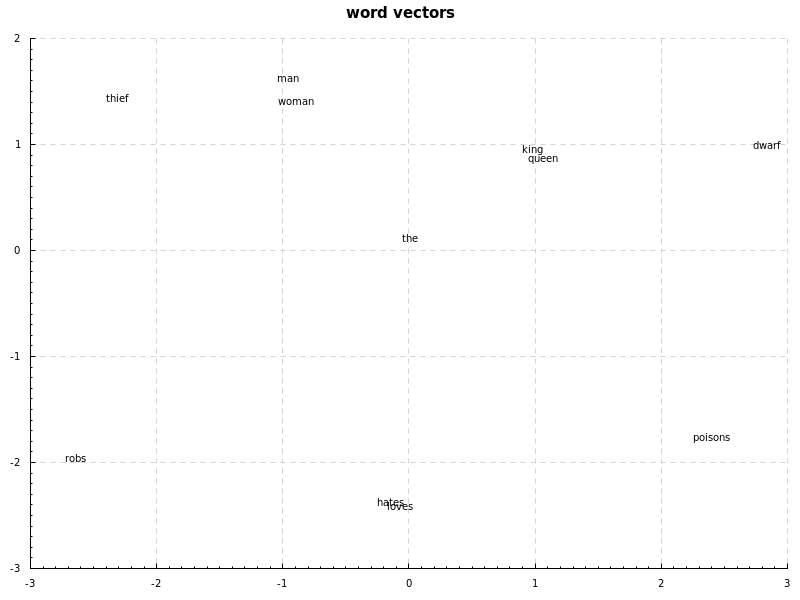موضوع‌ها
۱
فورک‌ها
۱
ستاره‌ها
۳۵
تاریخ ایجاد
۲۷ تیر ۱۳۹۵
آخرین بروزرسانی
حدود ۵ سال قبل
لایسنس

# sibe

A simple Machine Learning library.

## Simple neural network

``````import Numeric.Sibe

let a = (sigmoid, sigmoid') -- activation function
-- random network, seed 0, values between -1 and 1,
-- two inputs, two nodes in hidden layer and a single output
rnetwork = randomNetwork 0 (-1, 1) 2 [(2, a)] (1, a)

-- inputs and labels
inputs = [vector [0, 1], vector [1, 0], vector [1, 1], vector [0, 0]]
labels = [vector , vector , vector , vector ]

-- define the session which includes parameters
session = def { network = rnetwork
, learningRate = 0.5
, epochs = 1000
, training = zip inputs labels
, test = zip inputs labels
, drawChart = True
, chartName = "nn.png" -- draws chart of loss over time
} :: Session

initialCost = crossEntropy session

-- you can also use `sgd`, see the notmnist example
newsession <- run gd session

let results = map (`forward` newsession) inputs
rounded = map (map round . toList) results

cost = crossEntropy newsession

putStrLn \$ "- initial cost (cross-entropy): " ++ show initialCost
putStrLn \$ "- actual result: " ++ show results
putStrLn \$ "- rounded result: " ++ show rounded
putStrLn \$ "- cost (cross-entropy): " ++ show cost
``````

## Examples

``````# neural network examples
stack exec example-xor
stack exec example-424
# notMNIST dataset, achieves ~87.5% accuracy after 9 epochs
stack exec example-notmnist

# Naive Bayes document classifier, using Reuters dataset
# using Porter stemming, stopword elimination and a few custom techniques.
# The dataset is imbalanced which causes the classifier to be biased towards some classes (earn, acq, ...)
# to workaround the imbalanced dataset problem, there is a --top-ten option which classifies only top 10 popular
# classes, with evenly split datasets (100 for each), this increases F Measure significantly, along with ~10% of improved accuracy
# N-Grams don't seem to help us much here (or maybe my implementation is wrong!), using bigrams increases
# accuracy, while decreasing F-Measure slightly.
stack exec example-naivebayes-doc-classifier -- --verbose
stack exec example-naivebayes-doc-classifier -- --verbose --top-ten
``````

### notMNIST

notMNIST dataset, sigmoid hidden layer, cross-entropy loss, learning rate decay and sgd (`notmnist.hs`):notMNIST dataset, relu hidden layer, cross-entropy loss, learning rate decay and sgd (`notmnist.hs`):### Word2Vec

word2vec on a very small sample text:

``````the king loves the queen
the queen loves the king,
the dwarf hates the king
the queen hates the dwarf
the dwarf poisons the king
the dwarf poisons the queen
the man loves the woman
the woman loves the man,
the thief hates the man
the woman hates the thief
the thief robs the man
the thief robs the woman
``````

The computed vectors are transformed to two dimensions using PCA:

`king` and `queen` have a relation with `man` and `woman`, `love` and `hate` are close to each other, and `dwarf` and `thief` have a relation with `poisons` and `robs`, also, `dwarf` is close to `queen` and `king` while `thief` is closer to `man` and `woman`. `the` doesn't relate to anything.You can reproduce this result using these parameters:

``````let session = def { learningRate = 0.1
, batchSize = 1
, epochs = 10000
, debug = True
} :: Session
w2v = def { docs = ds
, dimensions = 30
, method = SkipGram
, window = 2
, w2vDrawChart = True
, w2vChartName = "w2v.png"
} :: Word2Vec
``````

This is a very small development dataset and I have to test it on larger datasets.